# Round Function In Excel – The Complete Guide

Microsoft Excel provides multiple formulas to round numbers. We have formulas like – ROUND, ROUNDUP, ROUNDDOWN, MROUND, INT, TRUNC, CEILING, FLOOR, FIXED, EVEN, ODD, and they all can be used to round numbers in excel.

Rounding is the process of removing the least significant digits from a number so that we are left with a simpler value that is very close to the original number.

In short, rounding gives you an approximate value that is quite accurate.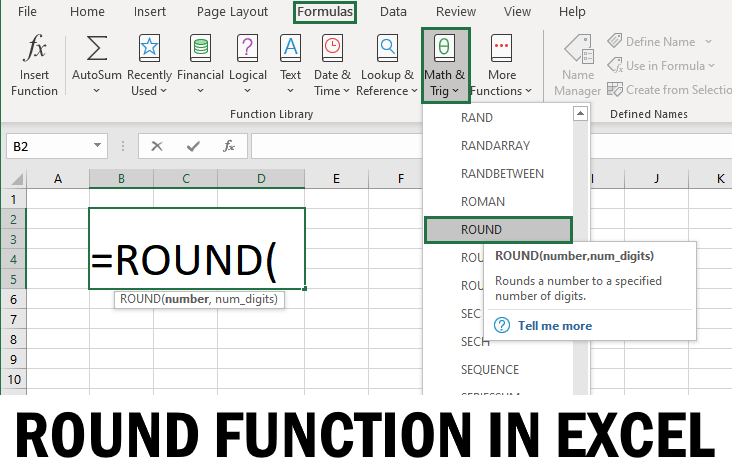## ROUND Function In Excel

The ROUND function is the most popular rounding function in excel. Round Function can round a number to the right or left of the decimal point.

### Syntax of Excel ROUND Function

=ROUND(number, num_digits)

Here, ‘number’ represents the value that needs to be rounded. This is a required parameter. This can be any real number; it can also be a reference to a cell containing a number.

The ‘num_digits’ argument is also a required parameter. This signifies the number of digits to which you want to round the number argument. This can take a value that is positive, negative, or zero.

1. If ‘num_digits’ is positive or greater than zero, the number is rounded to a specified number of decimal places.
2. If ‘num_digits’ is negative or less than zero, the number is rounded to the left of the decimal point.
3. If ‘num_digits’ is zero, the number is rounded to the nearest whole number or integer.

Note: If the number is already rounded to the given number of places, then no rounding occurs, and its value stays the same.

## Round to Right

In order to round the specified ‘number’ to the right of the decimal point, the ‘num_digits’ parameter should have a positive value.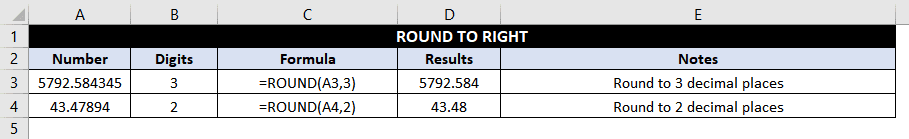### Example 1: Rounding a number to 3 decimal places.

Consider rounding the number 5792.584345 (present in the cell A3) to 3 decimal places.

The formula would be as follows:

=ROUND(A3, 3) //round to 3 decimal places

The result is 5792.584 since the number to the right of 4 is 3, so the rounding digit does not change.

### Example 2: Rounding a number to 2 decimal places.

Round the number 43.47894 (present in cell A4) to 2 decimal places.

The formula would be as follows:

=ROUND(A4, 2) //round to 2 decimal places

The result is 43.479 since the number to the right of 8 is 9, so the rounding digit is increased by one.

## Round to Left

In order to round the specified ‘number’ to the left of the decimal point, the ‘num_digits’ parameter should have a negative value.### Example 3: Rounding a number to the nearest 10.

We need to round the number 6593.578 (present in the cell A9) to the nearest 10.

The formula would be as follows:

=ROUND(A9,-1) //Round to the nearest 10

‘num_digits’ parameter, in this case, is -1, which means rounding needs to be done to the nearest 10, and hence the result is 6590.

### Example 4: Rounding a number to the nearest 100.

Round the number 5689.56 (present in cell A10) to the nearest 100.

The formula would be as follows:

=ROUND(A10,-2) //Round to the nearest 100

‘num_digits’ parameter, in this case, is -2, which means rounding needs to be done to the nearest 100, and hence the result is 5700.

Similarly, to round any number to the nearest 1000, the value of the ‘num_digits’ parameter should be -3 and so on.

### Example 5: Rounding a number to the nearest whole number.

Round the number 68.394 (present in cell A11) to the nearest whole number.

The formula would be as follows:

=ROUND(A11,0) //Round to the nearest integer

‘num_digits’ parameter, in this case, is 0, which means rounding needs to be done to the nearest 1, and hence the result is 68.

### Example 6: Rounding a negative number to the nearest integer.

Round the number -58.69 (present in the cell A12) to the nearest integer.

The formula for this problem would be as follows:

=ROUND(A12,0) //Round a negative number to the nearest integer

Like Example 5, in this case, the ‘num_digits’ parameter is 0, which means that the rounding needs to be done to the nearest 1, and hence the result is -58.

## Nesting Inside Round

Other functions that return a numeric result can be nested within the ROUND function. This can help in using the ROUND function as part of a more complicated formula.### Example 7: Round sum of two numbers

Round the result of the sum of two numbers 5869.548 and 5781.579 (present in cells A17and B17 respectively) to 1 decimal place.

The formula would be as follows:

=ROUND(A17+B17,1) //Round the sum of two numbers to 1 decimal place

The result of A17 +B17 = 11,651.127 and rounding it to 1 decimal place results in 11564.1.

### Example 8: Round the division quotient of 2 numbers

Round the division quotient of 2 numbers 669.547 and 61.578 (present in cells A18 and B18, respectively) to the nearest integer.

The formula would be as follows:

=ROUND(A18/B18,0) //Round the quotient of two numbers to the nearest integer

The quotient A18/B18 = 10.8731527493585, and rounding it to the nearest one results in 11.

## ROUNDDOWN Function In Excel

The ROUNDDOWN Function in Excel rounds down a given number to the specified number of decimal places.

The main difference between the ROUNDDOWN Function and ROUND Function is – In the ROUND function, the result depends on the rounding digit’s value. If it is less than five, then the number is rounded down; else, it is rounded up.

However, in the case of the ROUNDDOWN Function, the specified number is always rounded downwards.

### Syntax:

=ROUNDDOWN(number, num_digits)

Here, ‘number’ represents the number that needs to be rounded. This is a required parameter. This can be any real number; it can also be a reference to a cell containing a number.

The ‘num_digits’ argument is also a required parameter. This signifies the number of digits to which you want to round the number argument. This can take on a value that is positive, negative, or zero.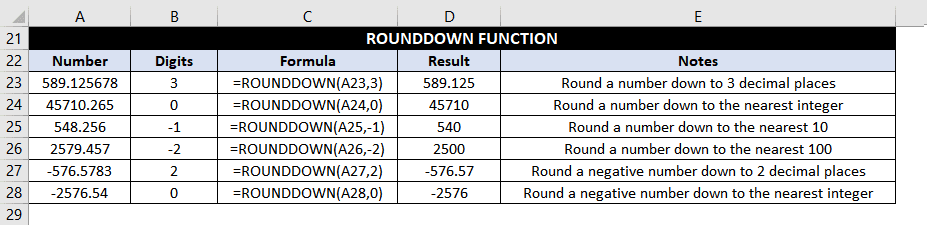### Example 9: Round a number down to 3 decimal places.

We want to round the number 589.125678 (present in cell A23) down to 3 decimal places.

The formula would be as follows:

=ROUNDDOWN(A23,3) //Round a number down to 3 decimal places

And the result is 569.125. Even if the rounding digit is followed by a number greater than 5, the rounding number is retained.

### Example 10: Round a number to the nearest integer.

Round a number 45710.265 (present in cell A24) down to the nearest integer.

The formula for this would be as follows:

=ROUNDDOWN(A24,0) //Round a number down to the nearest integer

Resulting in 45710.

### Example 11: Round a number down to the nearest 10.

Consider rounding the number 548.256 (present in cell A25) down to the nearest 10.

The formula would be as follows:

=ROUNDDOWN(A25,-1) //Round a number down to the nearest 10

And the result would be 540.

Note that even though the rounding number 4 is followed by a number greater than 5, however, ROUNDDOWN Function does not consider this and simply rounds down the number to the nearest 10.

### Example 12: Round a number down to the nearest 100.

In this example, we need to round the number 2579.457 (present in the cell A26) down to the nearest 100.

The formula for this would be as follows:

=ROUNDDOWN(A26,-2) //Round a number down to the nearest 100

Resulting in 2500. Even though the rounding number 5 is followed by 7, the number gets rounded down to 2500.

### Example 13:  Round a negative number down to 2 decimal places.

We have to round -576.5783 (present in cell A27) down to 2 decimal places.

The formula would be as follows:

=ROUNDDOWN(A27,2) //Round a negative number down to 2 decimal places

Resulting in a value -576.57.

### Example 14: Round a negative number down to the nearest integer.

So here we have to round -2576.54 (present in cell A28) down to the nearest integer.

The formula would be as follows:

=ROUNDDOWN(A28,0) //Round a negative number down to the nearest integer

Resulting in -2576.

In this case, ROUNDDOWN Function first finds the absolute value of the given number, which is 2576.54, then rounds it down to the nearest integer, which results in 2576. Finally, it affixes the negative sign, which gives -2576.

## ROUNDUP Function In Excel

The ROUNDUP function in Excel rounds up a given number to the specified number of decimal places. It is just opposite to the ROUNDDOWN Function.

The main difference between the ROUNDUP function and ROUND the Function is – In the ROUND function, the result depends on the rounding digit’s value. If it is greater than or equal to 5, then the number is rounded up; else, it is rounded down.

However, in the case of the ROUNDUP function, the specified number is always rounded upwards.

### Syntax:

=ROUNDUP (number, num_digits)

Here, ‘number’ represents the number that needs to be rounded. This is a required parameter. This can be any real number; it can also be a reference to a cell containing a number.

The ‘num_digits’ argument is also a required parameter. This signifies the number of digits to which you want to round the number argument. This can take on a value that is positive, negative, or zero.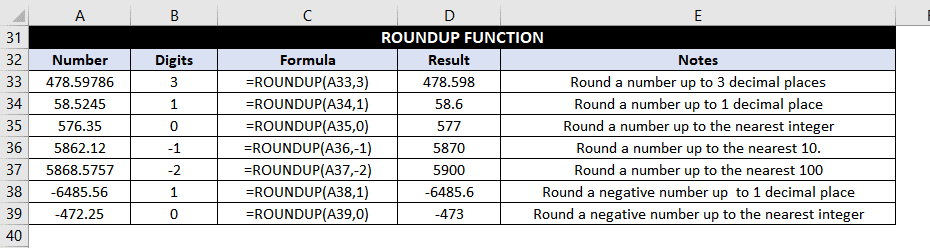### Example 15: Round a number up to 3 decimal places.

We need to round  478.59786 (present in cell A33) up to 3 decimal places in this example.

The formula would be as follows:

=ROUNDUP(A33,3) //Round a number up to 3 decimal places

Resulting in 478.598.

Here the rounding number 7 is increased by one, so it is replaced by the number 8 to give the result.

### Example 16: Round a number up to 1 decimal place.

Consider rounding a number 58.5245 (present in cell A34) up to 1 decimal place.

The formula for this would be as follows:

=ROUNDUP(A34,1) //Round a number up to 1 decimal place

And the result is 58.6.

Note that even though the rounding digit five is followed by 2, the value is not retained but increases by 1, resulting in 6 after the decimal point.

### Example 17: Round a number up to the nearest integer.

We need to round 576.35 (present in cell A35) up to the nearest integer.

The formula would be as follows:

=ROUNDUP(A35,0) //Round a number up to the nearest integer

Resulting in 577.

### Example 18: Round a number up to the nearest 10.

We need to round a number 5862.12 (present in cell A36) up to the nearest 10.

The formula would be as follows:

=ROUNDUP(A36,-1) //Round a number up to the nearest 10

And the result would be  5870.

In this example, rounding number 6 is rounded up to the nearest 10, thereby giving the result as 5870.

### Example 19: Round a number up to the nearest 100.

We need to round a number 5868.5757 (present in cell A37) up to the nearest 100.

The formula would be as follows:

=ROUNDUP(A37,-2) //Round a number up to the nearest 100

And the result is 5900. The rounding digit 8 is rounded up to the nearest 100, thereby giving the result as 5900.

### Example 20: Round a negative number to 1 decimal place.

We need to round a number -6485.56 (present in cell A38) up to 1 decimal place.

The formula would be as follows:

=ROUNDUP(A38,1) //Round a negative number up to 1 decimal place

And the result is -6485.6, the rounding digit 5 is increased by 1.

### Example 21: Round a negative number up to the nearest integer.

We need to round a number -472.25 present in cell A39 up to the nearest integer.

The formula would be as follows:

=ROUNDUP(A39,0) //Round a negative number up to the nearest integer

And the result is -473.

In this case, the ROUNDUP function first takes the absolute value of the number, which is 472.25, and rounds it up to the nearest integer, 473, then affixes the negative sign for the final result -473.

## MROUND Function In Excel

The MROUND Function is used for rounding a number to the nearest multiple. So, this Function will round a number up or down depending on the nearest multiple.

### Syntax:

=MROUND(number, multiple)

‘number’ signifies the number or the cell value that needs to be rounded.

‘multiple’ is the multiple you want to round your number to.

The last remaining digit can be rounded up (away from zero) or down (towards zero) depending on the remainder obtained by dividing the number by the multiple.

•        If the value of the remainder is equal to or greater than half of the multiple, then the MROUND Function rounds up the last digit.
•        If the value of the remainder is less than half the multiple, then the last digit is rounded down.

Let’s understand this with the help of some examples:### Example 22: Round a number to the nearest multiple of 2

In this example, we need to round 583 (present in the cell A44) to the nearest multiple of 2.

The formula for this would be as follows:

=MROUND(A44,2) //Round a number to the nearest multiple of 2

Now let’s try to find out the result – The nearest multiple of 2 can either be 584 or 582. Which one will it be?

If we divide the number (583) by the multiple (2), we get 291 as quotient and 1 as remainder. Since the remainder 1 is equal to half of the multiple (2) so, the number is rounded upwards to 584.

### Example 23: Round a number to the nearest multiple of 0.5

Let’s say we need to round a number 583.58 (present in cell A45) to the nearest multiple of 0.5.

The formula would be as follows:

=MROUND(A45,0.5) //Round a number to the nearest multiple of 0.5

Now let’s get to the result – The nearest multiple of 0.5 can either be 583.5 or 584.

If you divide 583.58 by 0.5, the quotient is 1167, and the remainder is 0.080, and since the remainder is less than half the multiple, so the final result is 583.5.

## FLOOR Function In Excel

The Excel FLOOR function is used for rounding a number down to the nearest specific multiple. It is similar to the Excel MROUND function except that it always rounds the number downwards.

### Syntax:

=FLOOR(number, significance)

‘number’ signifies the number or the cell value that needs to be rounded.

‘significance’ is the multiple you want to round your number to.

### Some rules for using the FLOOR function:

1. If both the ‘number’ and ‘significance’ are greater than 0, then the number is rounded down toward zero.
2. If the ‘number’ is positive, but ‘significance’ is negative, then the result will be an error #NUM.
3. If the ‘number’ is negative and ‘significance’ is positive, then the value is rounded away from zero.

Note: If you are using Excel 2003 and 2007, both the ‘number’ and significance’ must have the same sign, or else it will come out as an error. In later Excel versions, there is an improvement in the FLOOR function, and it can handle a negative number with a positive significance.### Example 24: Round a number down to the nearest multiple of 2.

Consider rounding a number 839 (present in cell A50) down to the nearest multiple of 2.

The formula would be as follows:

=FLOOR(A50,2) //Round a number down to the nearest multiple of 2

And the result would be 838 (not 840) since the number is rounded downwards.

### Example 25: Round a number down to the nearest multiple of 0.5

We need to round 9763.9 (present in cell A51) down to the nearest multiple of 0.5.

The formula would be as follows:

=FLOOR(A51,5) //Round a number down to the nearest multiple of 0.5

And the result is 9763.5

### Example 26: Round a negative number down to the nearest multiple of 3

Round the number -57894 (present in cell A52) to the nearest multiple of 3.

The formula would be as follows:

=FLOOR(A52,3) //Round a number down to the nearest multiple of 3

And the result is -57894 since the number is a multiple of 3; hence it is not rounded down.

### Example 27: Round a number down to the nearest multiple of -2.

Round the number 167 (present in the cell A53) to the nearest multiple of -2.

The formula would be as follows:

=FLOOR(A53,-2) //Round a number down to the nearest multiple of -2

Since the significance is negative and the number is positive, the result is #NUM error.

## CEILING Function In Excel

The Excel CEILING function is used for rounding a number upwards to the nearest specific multiple. It is similar to the Excel MROUND function except that it always rounds the number upwards.

### Syntax:

=CEILING(number, significance)

‘number’ signifies the number or the cell value that needs to be rounded.

‘significance’ is the multiple you want to round your number to.

Rounding with Excel CEILING function is similar to the FLOOR function except that the number is rounded up or away from zero instead of down.

### Some rules for using the CEILING function:

•        IF both arguments are positive, then the number is rounded up to the nearest multiple.
•        If the ‘number’ is positive, and the ‘significance’ is negative, the CEILING function will result in an error #NUM.
•        A negative ‘number’ with a positive ‘significance’ will also be rounded up.
•        If both the ‘number’ and ‘significance’ are negative, then the number is rounded down.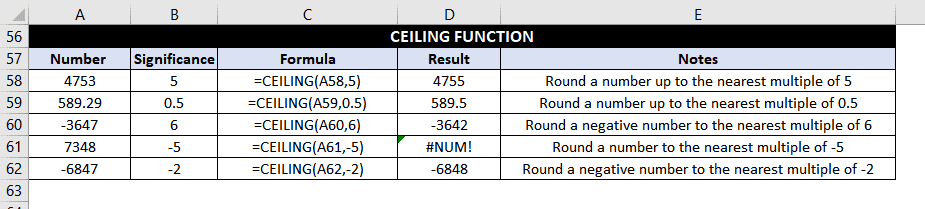### Example 28: Round a number up to the nearest multiple of 5

We need to round the number 4753 (present in the cell A58) up to the nearest multiple of 5.

The formula would be as follows:

=CEILING(A58,5) //Round a number up to the nearest multiple of 5

And the result is 4755 (not 4750) since the number is rounded up.

### Example 29: Round a number up to the nearest multiple of 0.5

Consider rounding a number 589.29 (present in the cell A59) up to the nearest multiple of 0.5.

The formula would be as follows:

=CEILING(A59,0.5) //Round a number up to the nearest multiple of 0.5

And the result is 589.5

### Example 30: Round a negative number up to the nearest multiple of 6.

Let’s say we need to round -3647 (present in the cell A60) up to the nearest multiple of 6.

The formula would be as follows:

=CEILING(A60,6) //Round a negative number to the nearest multiple of 6

And the result is -3642

### Example 31: Round a number up to the nearest multiple of -5

Let’s consider rounding a number 7348 (present in the cell A61) up to the nearest multiple of -5.

The formula for this would be as follows:

=CEILING(A61,-5) //Round a number to the nearest multiple of -5

And the result is the #NUM error since the CEILING function results in an error #NUM error if the ‘number’ is positive, and the ‘significance’ is negative.

### Example 32: Round a negative number to the nearest multiple of -2.

Round the number -6847 (present in the cell A62) to the nearest multiple of -2.

The formula would be as follows:

=CEILING(A62,-2) //Round a negative number to the nearest multiple of -2

And the result is -6848

## INT Function In Excel

The INT function is used to round down a number, with the result being an integer only. If a number is a decimal, then only the whole number is returned in the result.

Positive numbers are rounded down to zero, while negative numbers are rounded away from zero. Since the Function runs down, negative numbers become more negative.

This Function has only one argument, so it is the most straightforward Function to use out of all Excel’s Round functions.

### Syntax:

=INT(number)

‘number’ signifies the number or the cell value that needs to be rounded.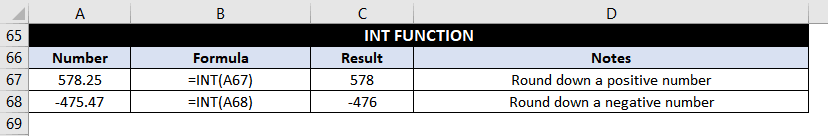### Example 33: Round down a positive number

Consider rounding a number 578.25 (present in the cell A67) using the INT Function.

The formula would be as follows:

=INT(A67) //Round down a positive number

And the result is 578

### Example 34: Round down a negative number

Round the number -475.47 (present in the cell A68) using the INT Function.

The formula would be as follows:

=INT(A68) //Round down a negative number

And the result is -476.

## TRUNC Function In Excel

TRUNC Function truncates a given number to a specified number of decimal places based on an optional argument. It does not round numbers but simply truncates as specified.

### Syntax:

=TRUNC(number, [num_digits])

‘number’ signifies the number or the cell value that needs to be truncated.

‘num_digits’ is an optional parameter which signifies the precision for truncation.

### Some rules for using the TRUNC Function:

•        If the ‘num_digits’ argument is not specified, then the number is rounded to an integer.
•        If the ‘num_digits’ argument is positive, then it truncates the specified number of digits to the right of the decimal point.
•        If the ‘num_digits’ argument is negative, then it truncates the specified number of digits to the left of the decimal point.

### TRUNC V/S INT

TRUNC and INT function are very similar because both can return the integer part of a number (when TRUNC is used without the optional argument).

However, from a logical point of view, the difference between the two functions is that – TRUNC simply truncates the decimal places from a number. At the same time, INT rounds the number down to an integer. So for positive numbers, the behavior of both TRUNC (without optional argument) and INT is the same.

However, with negative numbers, the results can be different. For instance – INT(-5.4) returns -6 because INT rounds down to the lower integer whereas TRUNC(-5.4) returns -5.### Example 34: Truncate a number 2 decimal places to the right

Truncate a number 879.5758 (present in the cell A73) to 2 decimal places to the right.

The formula would be as follows:

=TRUNC(A73,2) //Truncate a number 2 decimal places to the right

And the result is 879.57

### Example 35: Truncate a negative number 1 decimal to the left.

Truncate a number -257.68 (present in the cell A74) to 1 decimal to the left.

The formula would be as follows:

=TRUNC(A74,-1) //Truncate a number 1 decimal place to the left

And the result is -250.

### Example 36: Truncate the number to the nearest integer.

Truncate a number 6827.25 (present in the cell A75) to the nearest integer.

The formula would be as follows:

=TRUNC(A75,0) //Truncate a number to an integer

or

=TRUNC(A75) //Truncate a number to an integer

And the result is 6827.

## Even Function In Excel

Microsoft Even function rounds a given positive odd number up to the next even number. If the given number is odd and negative, then the number is rounded downwards (away from zero).

However, the given number is already an even number, so the number is retained as is.

### Syntax:

=EVEN(number)

‘number’ signifies the number or the cell value that needs to be rounded.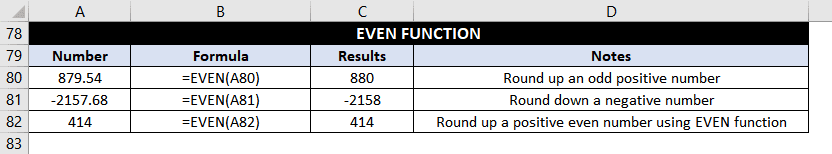### Example 37: Round up an odd positive number

Consider rounding a number 879.54 (present in the cell A80) using the EVEN Function.

The formula would be as follows:

=EVEN(A80) //Round up an odd positive number

And the result is 880 (rounded up)

### Example 38: Round up an odd negative number

Consider rounding a number -2157.68 (present in the cell A81) using the EVEN Function.

The formula would be

=EVEN(A81) //Round down a negative number

And the result is -2158 (rounded down)

### Example 38: Round up an even positive number

Consider rounding a number 414 (present in the cell A82) using the EVEN Function.

The formula would be as follows:

=EVEN(A82) //Round up a positive even number using EVEN function

And the result is 414 (since it is already an even number, so it remains the same)

## Odd Function In Excel

Microsoft ODD function rounds a given positive even number up to the next odd number. If the given number is even and negative, then the number is rounded downwards (away from zero).

However, the given number is already odd, so the number is retained as is.

### Syntax:

=ODD(number)

‘number’ signifies the number or the cell value that needs to be rounded.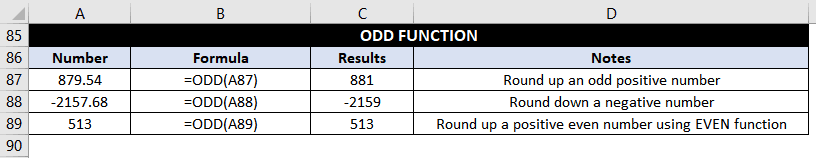### Example 37: Round up an even positive number

Consider rounding a number 879.54 (present in the cell A87) using the ODD Function.

The formula would be as follows:

=ODD(A87) //Round up an odd positive number

And the result is 881 (rounded up)

### Example 38: Round up an even negative number

Consider rounding a number -2157.68 (present in the cell A88) using the ODD Function.

The formula would be as follows:

=ODD(A88) //Round down a negative number

And the result is -2159 (rounded down)

### Example 38: Round up an odd positive number

Consider rounding a number 513 (present in the cell A89) using the ODD Function.

The formula would be as follows:

=ODD(A89) //Round up a positive even number using EVEN function

And the result is 513 (since it is already an odd number, so it remains the same)

So, these were all the Rounding Functions in Excel. Hope you would find them useful 🙂About Ankit Kaul

Ankit is the founder of Excel Trick. He is tech Geek who loves to sit in front of his square headed girlfriend (his PC) all day long. :D. Ankit has a strong passion for learning Microsoft Excel. His only aim is to turn you guys into 'Excel Geeks'.